## 6.4.1 Basic Definitions and Concepts

This section builds on the semi-algebraic model definitions from Section 3.1 and the polynomial definitions from Section 4.4.1. It will be assumed that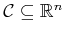, which could for example arise by representing each copy of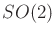or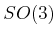in its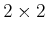or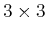matrix form. For example, in the case of a 3D rigid body, we know that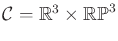, which is a six-dimensional manifold, but it can be embedded in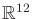, which is obtained from the Cartesian product of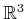and the set of allmatrices. The constraints that force the matrices to lie inorare polynomials, and they can therefore be added to the semi-algebraic models of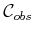and. If the dimension ofis less than, then the algorithm presented below is sufficient, but there are some representation and complexity issues that motivate using a special parameterization ofto make both dimensions the same while altering the topology ofto become homeomorphic to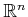. This is discussed briefly in Section 6.4.2.

Suppose that the models inare all expressed using polynomials from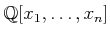, the set of polynomials6.6 over the field of rational numbers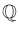. Let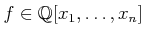denote a polynomial.

Subsections
Steven M LaValle 2012-04-20